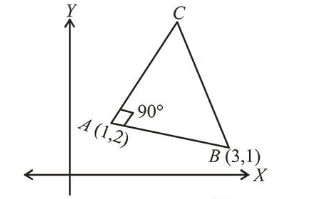# A triangle A B C lying in the first quadrantQuestion:

A triangle $A B C$ lying in the first quadrant has two vertices as $A(1,2)$ and $B(3,1)$. If $\angle B A C=90^{\circ}$, and ar $(\triangle A B C)=5 \sqrt{5}$ sq. units, then the abscissa of the vertex $C$ is:

1. (1) $1+\sqrt{5}$

2. (2) $1+2 \sqrt{5}$

3. (3) $2+\sqrt{5}$

4. (4) $2 \sqrt{5}-1$

Correct Option: , 2

Solution:

Let $\triangle A B C$ be in the first quadrant

Slope of line $A B=-\frac{1}{2}$

Slope of line $A C=2$

Length of $A B=\sqrt{5}$It is given that $\operatorname{ar}(\triangle A B C)=5 \sqrt{5}$

$\therefore \frac{1}{2} A B \cdot A C=5 \sqrt{5} \Rightarrow A C=10$

$\therefore$ Coordinate of vertex $C=(1+10 \cos \theta, 2+10 \sin \theta)$

$\because \tan \theta=2 \Rightarrow \cos \theta=\frac{1}{\sqrt{5}}, \sin \theta=\frac{2}{\sqrt{5}}$

$\therefore$ Coordinate of $\mathrm{C}=(1+2 \sqrt{5}, 2+4 \sqrt{5})$

$\therefore$ Abscissa of vertex $C$ is $1+2 \sqrt{5}$